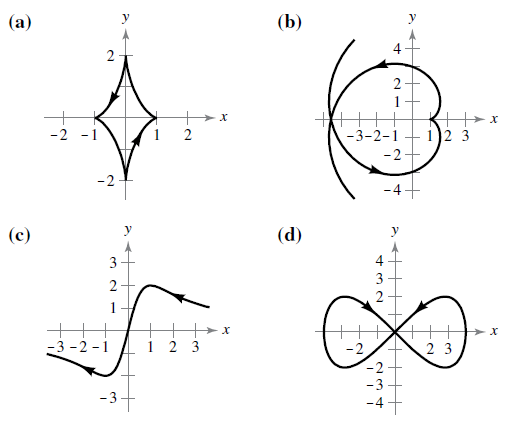Chapter 10.2, Problem 72E

Chapter
Section
Textbook Problem

Matching In Exercises 71–74, match the set of parametric equation with its graphs. [The graphs are labeled (a), (b), (c), and (d).] Explain your reasoning. Evolute of ellipse : x = cos 3 θ , y = 2 sin 3 θTo determine
Match the curve with the given equation

Explanation

Given: x=cos3θ,y=2sin3θ

Explanation: We plot the graph on Wx Maxima by using the following steps

Step 1: Open Wx Maxima

Step2: Click on the plot section on the toolbar

Step3: Click on the plot 2d section under the plot toolbar

Step 4: Click on parametric which appears after clicking on special button on the right of the bar

Still sussing out bartleby?

Check out a sample textbook solution.

See a sample solution

The Solution to Your Study Problems

Bartleby provides explanations to thousands of textbook problems written by our experts, many with advanced degrees!

Get Started

Simplify the expressions in Exercises 97106. x3/2x5/2

Finite Mathematics and Applied Calculus (MindTap Course List)

In Exercises 47-52, find and simplify f(a+h)f(a)h (h 0) for each function. 47. f(x) = x2 + 1

Applied Calculus for the Managerial, Life, and Social Sciences: A Brief Approach

In Problems 1 and 2, factor out the common monomial factor. 1.

Mathematical Applications for the Management, Life, and Social Sciences